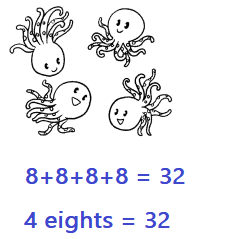Go through the Math in Focus Grade 1 Workbook Answer Key Chapter 18 Practice 1 Adding the Same Number to finish your assignments.

Count the number of groups. Then count the number of bugs in each group. Write the numbers in the blanks.

Example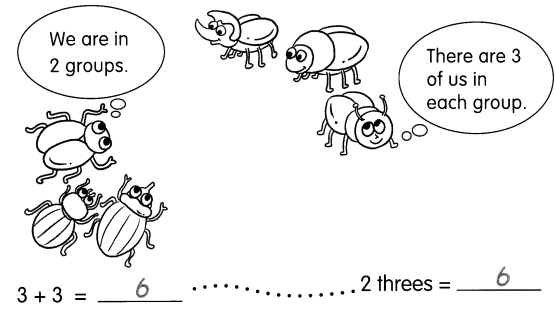Question 1.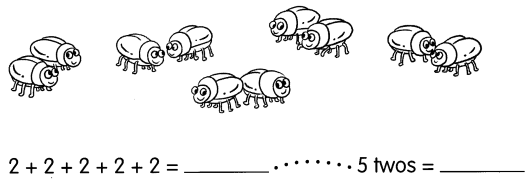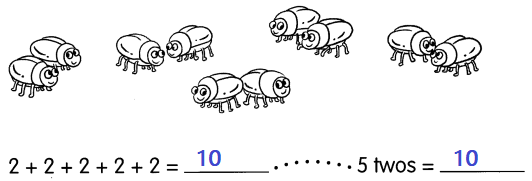Explanation:
Count the number of groups.
Then count the number of bugs in each group.
Similarly, multiplying 5 x 2 = 10

Count the number of groups. Then count the number of bugs in each group. Write the numbers in the blanks.

Question 2.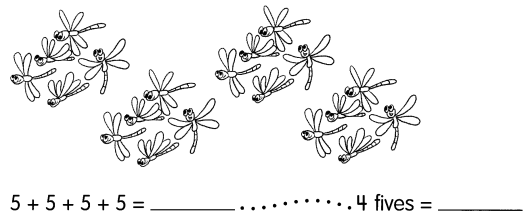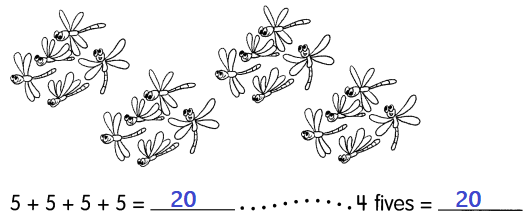Explanation:
Count the number of groups.
Then count the number of dragon flies in each group.
Similarly, multiplying 4 x 5 = 20

Question 3.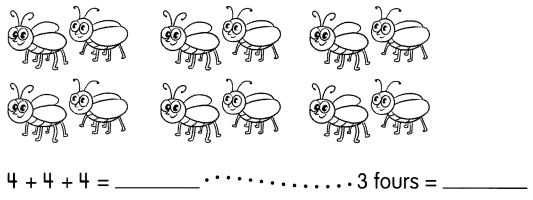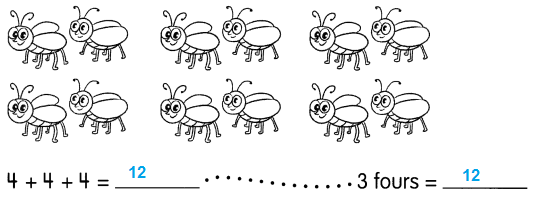Explanation:
Count the number of groups.
Then count the number of bugs in each group.
Add 4 + 4+ 4 = 12
Similarly, multiplying 4 x 3 = 12

Question 4.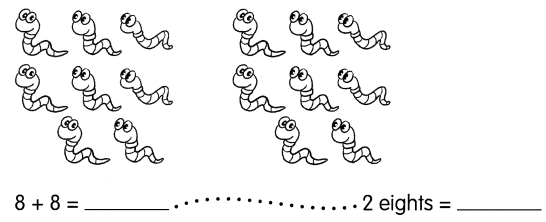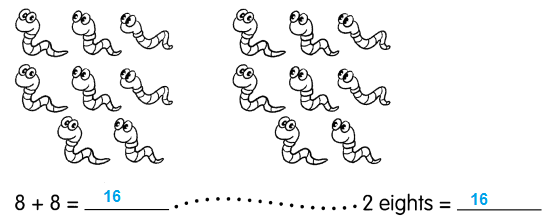Explanation:
Count the number of groups.
Then count the number of caterpillars in each group.
Add 8 + 8 = 16
Similarly, multiplying 8 x 2 = 16

Look at the pictures. Then fill in the blanks.

Question 5.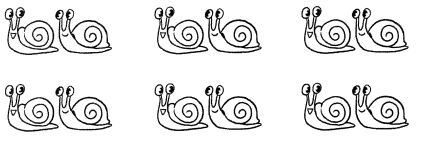_________ + _________ + _________ + _________ + _________ + _________
= _________
_________ twos = _________
There are _________ snails in all.
Explanation:
There are 12 snails in all.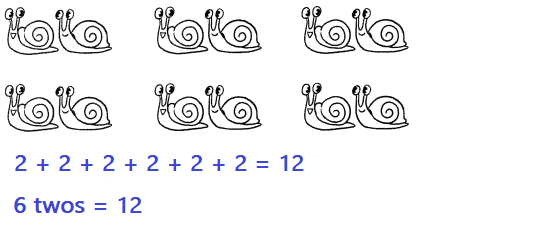Question 6.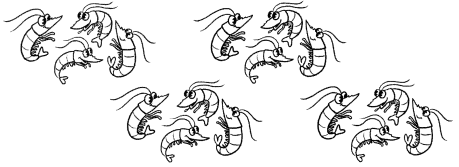_________ + _________ + _________ + _________ = _________
_________ fours = _________
There are _________ shrimps in all.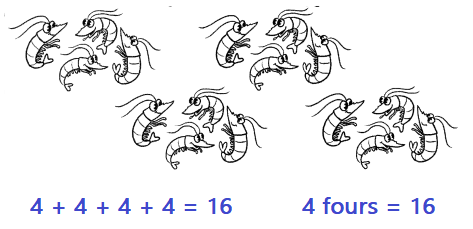There are 16 shrimps in all.
Explanation:
Count the number of groups.
Then count the number of shrimps in each group.
4 x 4 = 16

Look at the picture. Then fill in the blanks.

Example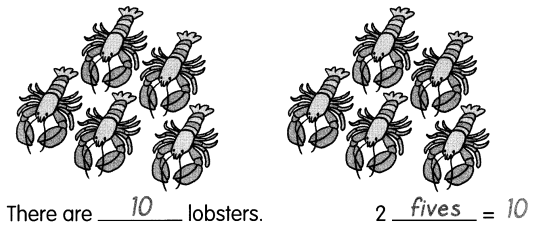Question 7.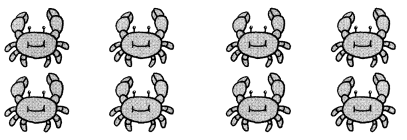There are __________ crabs
4 ____________ = ____________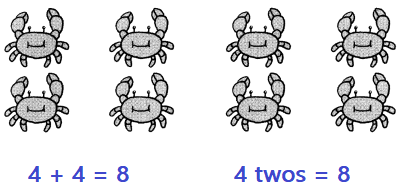there are 8 crabs
Explanation:
Count the number of groups.
Then count the number of crabs in each group.
2 x 4 = 8

Question 8.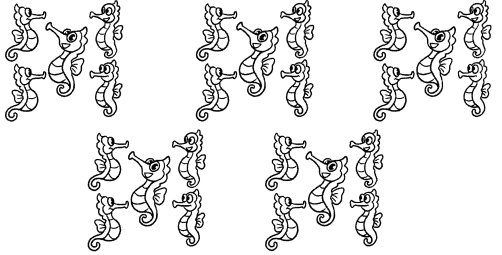There are __________ seahorses
5 ____________ = ____________
Explanation:
There are 5 groups of seahorses.
Each group has 5 seahorses.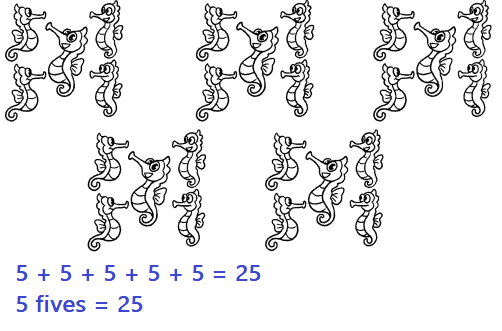Look at the picture. Then fill in the blanks.

Question 9.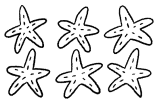A starfish has _________ arms.
6 five = ___________
6 starfishes have ___________ arms.
Explanation:
There are 6 star fishes,
each fish has 5 arms.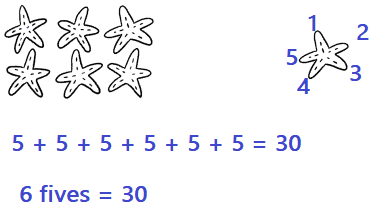Question 10.
Each dress has 5 buttons.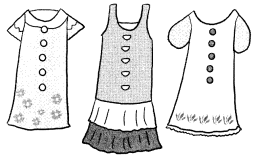3 ___________ = ____________
3 dresses have ____________ buttons
Explanation:
There are 3 dresses, each dress has 5 buttons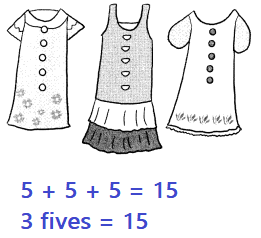Question 11.
One flower has ___________ petals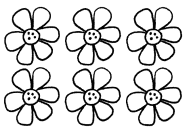6 ___________ = ___________
6 flowers have ___________ petals.
Explanation:
There are 6 flowers.
Each flower has 6 petals.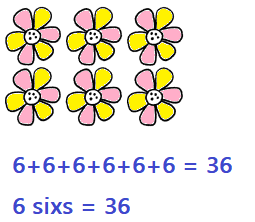Look at the picture. Then fill in the blanks.

Question 12.
Each plant has ___________ leaves.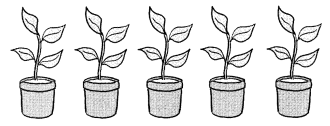5___________ = ___________
5 plants have ___________ leaves.
Explanation:
There are 5 pants.
Each plant has 4 leaves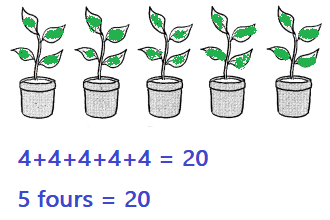Question 13.
An octopus has ___________ arms.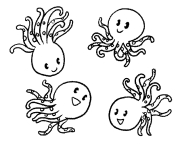4 ___________ = ___________
4 octopuses have ___________ arms.